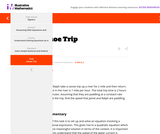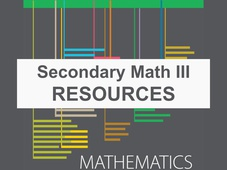# Secondary Mathematics III Resources

This collection contains highly recommended Secondary Mathematics IIIlessons, activities, and other resources from the eMedia library.

267 affiliated resources

# Search Resources

View
Selected filters: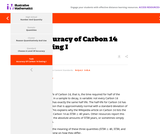Restricted Use
Rating

This task examines, from a mathematical and statistical point of view, how scientists measure the age of organic materials by measuring the ratio of Carbon 14 to Carbon 12. The focus here is on the statistical nature of such dating.

Subject:
Mathematics
Material Type:
Activity/Lab
Provider:
Illustrative Mathematics
04/26/2022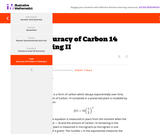Restricted Use
Rating

This Illustrative Mathematics task is a refinement of "Carbon 14 dating" which focuses on accuracy. While the mathematical part of this task is suitable for assessment, the context makes it more appropriate for instructional purposes. This type of question is very important in science and it also provides an opportunity to study the very subtle question of how errors behave when applying a function: in some cases the errors can be magnified while in others they are lessened.

Subject:
Mathematics
Material Type:
Activity/Lab
Provider:
Illustrative Mathematics
04/26/2022Unrestricted Use
CC BY
Rating

This Teaching Channel video illustrates how two teachers' collaboration impacted their algebra program. This site provides a lesson plan and student handouts. (10 min.)

Provider:
Teaching Channel
06/22/2022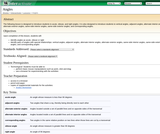Unrestricted Use
CC BY
Rating

Students are introduced to all kinds of angles in this lesson plan, including acute, obtuse, right, vertical, adjacent, and corresponding among others.

Provider:
Interactive
06/22/2022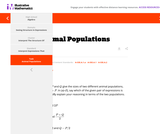Restricted Use
Rating

This Illustrative Mathematics task students have to interpret expressions involving two variables in the context of a real world situation.

Subject:
Mathematics
Material Type:
Activity/Lab
Provider:
Illustrative Mathematics
04/26/2022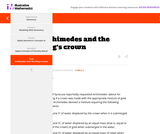Unrestricted Use
CC BY
Rating

This problem combines the ideas of ratio and proportion within the context of density of matter.

Provider:
Illustrative Mathematics
06/22/2022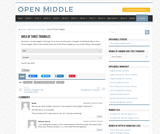Restricted Use
Rating

Open Middle provides math problems that have a closed beginning, a closed end, and an open middle. This means that there are multiple ways to approach and ultimately solve the problems. Open middle problems generally require a higher Depth of Knowledge than most problems that assess procedural and conceptual understanding.

Subject:
Mathematics
Material Type:
Activity/Lab
Provider:
Open Middle Math
04/26/2022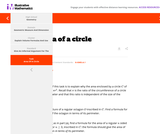Unrestricted Use
CC BY
Rating

This purpose of this task is to develop an understanding of the formula for the area of the circle.

Provider:
Illustrative Mathematics
06/22/2022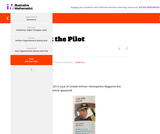Unrestricted Use
CC BY
Rating

This task can be used as a classroom activity. There is a lot of opportunity to discuss the process of mathematical modeling. It serves to illustrate MP 4 - Model with Mathematics, not just by engaging in the practice, but also by investigating what this practice entails.

Provider:
Illustrative Mathematics
06/22/2022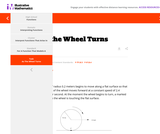Restricted Use
Rating

In this task, students use trigonometric functions to model the movement of a point around a wheel and, in the case of part (c), through space (F-TF.5). Students also interpret features of graphs in terms of the given real-world context (F-IF.4).

Subject:
Mathematics
Material Type:
Activity/Lab
Provider:
Illustrative Mathematics
04/26/2022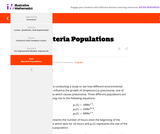Unrestricted Use
CC BY
Rating

This task provides a real world context for interpreting and solving exponential equations. There are two solutions provided for part (a). The first solution demonstrates how to deduce the conclusion by thinking in terms of the functions and their rates of change. The second approach illustrates a rigorous algebraic demonstration that the two populations can never be equal.

Provider:
Illustrative Mathematics
06/22/2022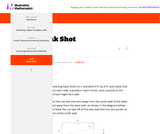Unrestricted Use
CC BY
Rating

This task asks students to use similarity to solve a problem in a context that will be familiar to many, though most students are accustomed to using intuition rather than geometric reasoning to set up the shot.

Provider:
Illustrative Mathematics
06/22/2022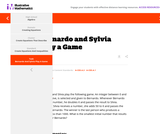Unrestricted Use
CC BY
Rating

This task presents a simple but mathematically interesting game whose solution is a challenging exercise in creating and reasoning with algebraic inequalities. The core of the task involves converting a verbal statement into a mathematical inequality in a context in which the inequality is not obviously presented, and then repeatedly using the inequality to deduce information about the structure of the game.

Provider:
Illustrative Mathematics
06/22/2022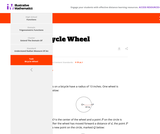Unrestricted Use
CC BY
Rating

The purpose of this task is to introduce radian measure for angles in a situation where it arises naturally.

Provider:
Illustrative Mathematics
06/22/2022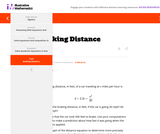Unrestricted Use
CC BY
Rating

The purpose of this task is to give an application arising from a real-world situation in which a quadratic equation arises, and where it is natural to use a graphical method to find an approximate solution and the quadratic formula to find an exact solution

Provider:
Illustrative Mathematics
06/22/2022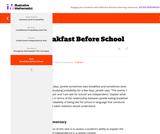Unrestricted Use
CC BY
Rating

The purpose of this task is to assess a student's ability to explain the meaning of independence in a simple context.

Provider:
Illustrative Mathematics
06/22/2022Unrestricted Use
CC BY
Rating

This task can be used as an instructional task to develop students understanding of independence and students ability to calculate the probability of intersection events using the multiplication rule for independent events.

Provider:
Illustrative Mathematics
06/22/2022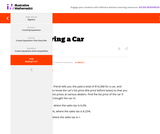Unrestricted Use
CC BY
Rating

The emphasis in this task is not on complex solution procedures. Rather, the progression of equations, from two that involve different values of the sales tax, to one that involves the sales tax as a parameter, is designed to foster the habit of looking for regularity in solution procedures, so that students don't approach every equation as a new problem but learn to notice familiar types.

Provider:
Illustrative Mathematics
06/22/2022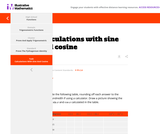Unrestricted Use
CC BY
Rating

The goal of this task is to study some values of sinx and cosx looking for and then explaining patterns.

Provider:
Illustrative Mathematics